1.
A man standing at a point P is watching the top of a tower, which makes an angle of elevation of 30° with the man's eye. The man walks some distance towards the tower to watch its top and the angle of elevation becomes 60°. What is the distance between the base of the tower and the point P?
•  A. 4 3 units
• C.
12 units
• E.
None of these
• B.
8 units
• D.
• Answer & Explanation
• Report
Explanation :
 One of AB, AD and CD must have been given. So, the data is inadequate.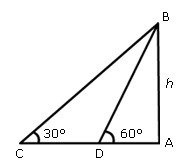Report
 Name Email
2.
A man on the top of a vertical observation tower observers a car moving at a uniform speed coming directly towards it. If it takes 12 minutes for the angle of depression to change from 30° to 45°, how soon after this will the car reach the observation tower?
• A.
14 min, 35 sec.
• C.
16 min. 23 s.
• B.
15 min 49 sec.
• D.
18 min. 5 sec.
• Answer & Explanation
• Report
Explanation :
Let AB be the tower and C and D be the two positions of the car.
 Then,ACB = 45°,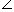ADB = 30°
Let AB = h, CD = x and AC = y.
 AB AC
= tan 45° = 1
 h y
= 1   y = h.
= tan 30° =
 1 3

 h x + y
=
 1 3

 x + y = 3 h
x = (x + y) - y = 3  h - h = h( 3  - 1)
 Now, h( 3 - 1) is covered in 12 min.
So, h will be covered in
12
 h( 3 - 1)
x h   =
12
 ( 3 - 1)
min
=
 1200 73
min. = 16 min. 23 sec.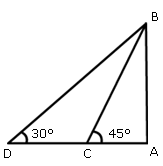Report
 Name Email
3.
The angle of elevation of a ladder leaning against a wall is 60° and the foot of the ladder is 4.6 m away from the wall. The length of the ladder is:
• A.
2.3 m
• C.
7.8 m
• B.
4.6 m
• D.
9.2 m
• Answer & Explanation
• Report
Explanation :
Let AB be the wall and BC be the ladder.
 Then,ACB = 60° and AC = 4.6 m.

 AC BC
= cos 60° =
 1 2

BC = 2 x AC = (2 x 4.6) m = 9.2 m.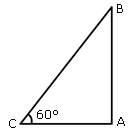Report
 Name Email
4.
A man is watching from the top of a tower a boat speeding away from the tower. The boat makes an angle of depression of 45° with the man�s eye when at a distance of 60 metres from the tower. After 5 seconds, the angle of depression becomes 30°. What is the approximate speed of the boat, assuming that it is running in still water?
• A.
32 kmph
• B.
36 kmph
• C.
38 kmph
• Answer & Explanation
• Report
Explanation :
Let AB be the tower and C and D be the two positions of the boats.
 Then,ACB = 45°,ADB = 30° and AC = 60 m.
Let AB = h.
Then,
 AB AC
= tan 45° = 1   AB = AC   h = 60 m.

And,
= tan 30° =
 1 3

 AD = (AB x 3 ) = 60 3 m

CD = (AD - AC) = 60( 3  - 1) m.
Hence, required speed =
 60 ( 3 - 1)
5
m/s = (12 x 0.73) m/s
12 x 0.73 x
 18 5
km/hr = 31.5 km/hr
 = 32 km/hr.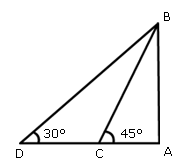Report
 Name Email
5.
From a point P on a level ground, the angle of elevation of the top of a tower is 30°. If the tower is 100 m hight, the distance of point P from the foot of the tower is:
• A.
149 m
• C.
173 m
• B.
156 m
• D.
200 m
• Answer & Explanation
• Report
Explanation :
 Let AB be the tower. Then,APB = 30° and AB = 100 m
 AB AP
= tan 30° =
 1 3

 AP = (AB x 3 ) = 100 3 m

 = (100 x 1.73) m = 173 m.Report
 Name Email
• Pages
• 1
• 2